Grade calculator is a free online tool to calculate weighted average grades. Also, it supports percentage, letter, and point grades.

Related

Student life is literary full of grades, marks, and percentages. If you are in school or college then grade calculator will surely help you by calculating your class or semester grades. Also, you can know your subject’s abilities using this tool. Even more, it helps you to set up goals for the next exam preparation.You can use your mobile or computer calculator for calculating grades manually. But it’s not a convenient way to do so. Also, it will take a lot of time to figure out. Most importantly, no one can guarantee that the end result is true or not. That’s why it is recommended to use our grade calculator to make this task easier and faster. Also, it will never give false results. Because it is working on programmed algorithms.

### Definition, Formula & Calculation

Firstly, let’s discuss the definition of grade calculation.

Definition: The weighted grade is equal to the sum of weight(W) in percentage(%) multiply by grades(G). So, the formula will be like this:

W1 × G1 + W2 × G2 + W3 × G3 + W4 × G4 + W5 × G5 + …

Now let’s take some examples to understand it more deeply.

#### Example 1:

Assume that:

Math course with a grade of 90 and a weight of 20%.
Physics course with a grade of 80 and a weight of 40%.
Social Science course with a grade of 85 and a weight of 25%.

So, the weighted grade calculation is as below:

w1 × g1 + w2 × g2 + w3 × g3 =
(20% × 90) + (40% × 80) + (25% × 85) =
18 + 32 + 21.25 =
71.25

Now, if the weights are not in percentage (if it’s in hours or points) then we need to divide achieved grades by the sum of all weights.

Therefore, the calculation for these three subjects will look like this:

(W1 × G1 + W2 × G2 + W3 × G3)
(W1 + W2 + W3)

So, the final calculation is:
(20×90) + (40×80) + (25×85) / (20 + 40 + 25)
= 7125 / 85 = 83.82

#### Example 2:

Let’s take:

5 points Math course with a grade of 90.
4 points Physics course with a grade of 85.
2 points Social Science course with a grade of 75.

(W1 × G1 + W2 × G2 + W3 × G3) / (W1 + W2 + W3)
(5×90 + 4×85 + 2×75) / (5 + 4 + 2) =
(450 + 336 + 150) / 11 =
85.09

Above all calculations are used to calculate your exam or semester grades. But it’s complex when you do it manually. So, it’s better to use the grade calculator to make it faster and easier.

### Uses

Most importantly, you must have to set your aim to do more productive preparation. Therefore, aim for the grade that you want and use our calculator to track the results. Also, you can know what needs to achieve your aim. In addition, it will also motivate you to study harder.

#### How to use Grade Calculator?

• Firstly, it’s an online service. So, make sure you have a device with a proper Internet connection.
• Then open any browser like chrome, safari, or firefox and visit the Grade Calculator.
• When it completely loads up, you will see some empty fields.
• So, enter the grade and weight values one by one as needed.
• In addition, it supports percentage, letters, and points grade calculation. So, you can select any one of them.
• After entering values, just click on the “Calculate” button.

Are you still confused about how to use the calculator? Here are the step-by-step instructions with screenshots that you can follow and do the calculation easily.

##### Setp 1: Choose the Calculation Type

Firstly, choose which type of calculation you want to do. There are three options: Percentages, Letters, and Points.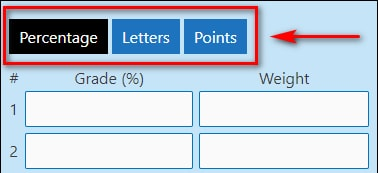##### Step 2: Enter the Values

Now the second step is entering the values. Most importantly, you have to enter the values as per the selected calculation type.

If you choose the Percentage then you have to enter Grade(%) and Weight.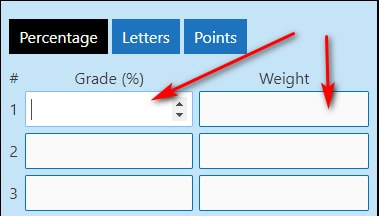If you are calculating Letters then firstly, you can select the grade from dropdown options and then enter the credits.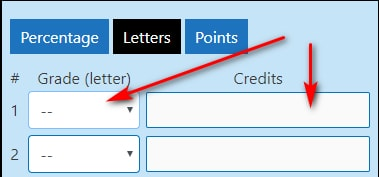For Points calculation, you can enter Grade(in points) and Max Grade(it’s optional).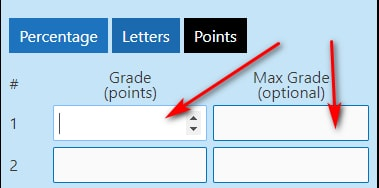##### Step 3: Enter Goal or Required Grade

Another useful option in the grade calculator is you can set your goal grade. So, you can find how much more grade is required to reach your goal. But it’s optional. So, you can leave it if you don’t want to use it. However, if you use it then you can see the additional grade needed to reach the aim grade.##### Step 4: Calculate

Lastly, you can press the “Calculate” button to get the final result on your screen. Also, you can add rows by entering the “Add Row” button for more subjects.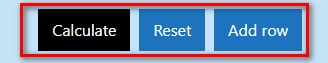##### Step 5: Get Output

Finally, you will get the output with a bar chart. Even more, you will get a GPA for letters calculation and Total Grades in points calculation.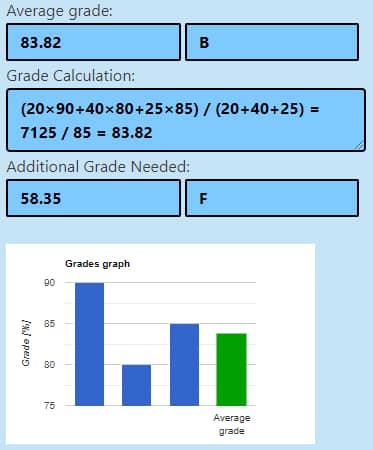So, now you can understand the proper way to use the calculator. Use it to check your grade and share it with your friends.